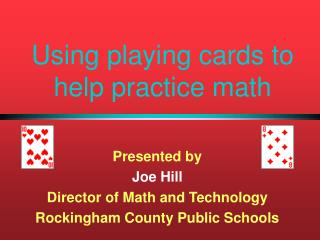Download PresentationUsing playing cards to help practice math

# Using playing cards to help practice math - PowerPoint PPT PresentationDownload Presentation## Using playing cards to help practice math

- - - - - - - - - - - - - - - - - - - - - - - - - - - E N D - - - - - - - - - - - - - - - - - - - - - - - - - - -
##### Presentation Transcript

1. Using playing cards to help practice math Presented by Joe Hill Director of Math and Technology Rockingham County Public Schools

2. Introduction... Here are some ideas on how you can practice math skills using common playing cards.

3. SOLUTION PROBLEM Try this card activity... Arrange the five cards shown below in the five boxes shown to the right such that the sum, both horizontally and vertically, is 8.

4. SOLUTION PROBLEM Now change it a little…. Arrange the five cards shown below in the five boxes shown to the right such that the sum, both horizontally and vertically, is 9.

5. SOLUTION PROBLEM And change it again... Arrange the five cards shown below in the five boxes shown to the right such that the sum, both horizontally and vertically, is 10.

6. SOLUTION PROBLEM Try this card activity Arrange the six cards shown below in the six boxes shown to the right such that the sum along all three arrows is9.

7. SOLUTION PROBLEM Now change the sum... Arrange the six cards shown below in the six boxes shown to the right such that the sum along all three arrows is 10.

8. SOLUTION PROBLEM Change it again... Arrange the six cards shown below in the six boxes shown to the right such that the sum along all three arrows is 11.

9. SOLUTION PROBLEM And again... Arrange the six cards shown below in the six boxes shown to the right such that the sum along all three arrows is 12.

10. PROBLEM SOLUTION A new challenge Place the eight cards in the eight boxes so that no two consecutive cards are adjacent in any way--including corners.

11. SOLUTION PROBLEM Next challenge….deal the cards Begin with the ten cards 1, 2, 3, 4, 5, 6, 7, 8, 9, and 10. Deal them in this manner: deal the first one, then put the second one on the bottom of the deck. Deal the third card, then put the fourth one on the bottom of the deck. Continue in this fashion until all are dealt. Arrange the cards so that they will be dealt in order: 1, 2, 3, 4, 5, 6, 7, 8, 9, and 10.

12. The End! Hope you enjoyed solving problems with playing cards.

13. SUM = 8 SOLUTION Notice the middle card Return

14. SUM = 9 SOLUTION Notice the middle card. See a pattern? Return

15. SUM = 10 SOLUTION Notice the middle card. See a pattern? Return

16. SUM = 23 SOLUTION 23 is the lowest sum possible for this game. Other possible sums are 24 and 25. Return

17. ALGEBRA SOLUTION For any five consecutively numbered cards, you can find sums of (3n + 5), (3n + 6), and (3n + 7) where n is the lowest card. In the example below, the possible sums are 17, 18, and 19. Return

18. SUM = 9 SOLUTION Notice the numbers in the corners. Return

19. SUM = 10 SOLUTION Notice the numbers in the corners. See a pattern? Return

20. SUM = 11 SOLUTION Notice the numbers in the corners. See a pattern? Return

21. SUM = 12 SOLUTION Could you make a sum of 13 all three ways?? Return

22. SUM = 21 SOLUTION What other sums could you make with these six cards? 22, 23, and 24 Return

23. SOLUTION Notice the middle numbers. Return

24. CARD DEAL SOLUTION Start with ten blanks: __ __ __ __ __ __ __ __ __ __ Fill in the first five: 1 __ 2 __ 3 __ 4 __ 5 __ Count the __ as “bottom of the deck” and skip all previously dealt cards: 1 6 2 10 3 7 4 9 5 8 Return